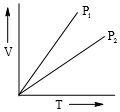At moderate pressure, the Vander wall's equation become:-

1. PVm = RT

2. P(Vm-b) = RT

3. (P+a/V2m) (Vm )= RT

4. P = RT/Vm + a/V2m

Concept Questions :-

Vanderwaal Correction
High Yielding Test Series + Question Bank - NEET 2020

Difficulty Level:

The compressibility factor for a real gas at high pressure is:-

1. 1

2. 1+Pb/RT

3. 1-Pb/RT

4. 1-RT/Pb

Concept Questions :-

Compressibility Factor
High Yielding Test Series + Question Bank - NEET 2020

Difficulty Level:

A gas diffuses four time quickly as oxygen. The molecular weight of gas is:

1. 2

2. 4

3. 8

4. 16

Concept Questions :-

Graham's Law
High Yielding Test Series + Question Bank - NEET 2020

Difficulty Level:

Which of the following mixtures of gases at room temperature does not obey Dalton's law of partial pressure?

(1) He and O2

(2) NH3 and HCL

(3) H2 and He

(4) N2 and He

High Yielding Test Series + Question Bank - NEET 2020

Difficulty Level:

1.6g O2 gas and 0.1g H2 gas are placed in a 1.12 L flask at 00c then find out total pressure of gas mixture:

1. 2 atm

2. 3 atm

3. 4 atm

4. 1 atm

Concept Questions :-

Ideal Gas Law
High Yielding Test Series + Question Bank - NEET 2020

Difficulty Level:

Rate of diffusion of LPG (a mixture of n-butane and propane) is 1.25 times of SO3. Hence, mass fraction of n-butane in LPG is

1. 0.75

2. 0.25

3. 0.50

4. 0.67

Concept Questions :-

Graham's Law
High Yielding Test Series + Question Bank - NEET 2020

Difficulty Level:

An open flask contining air is heated from 300 K to 500 K. What percentage of air will be escaped to the atmosphere, if pressure is keeping constant?

1. 80

2. 40

3. 60

4. 20

Concept Questions :-

Ideal Gas Law
High Yielding Test Series + Question Bank - NEET 2020

Difficulty Level:

V versus T curves at constant pressure P1 and P2 for an ideal gas are shown in figure. Which is correct?(1)P1>P2

(2)P1<P2

(3)P1=P2

(4)All of these

Concept Questions :-

Gas Law
High Yielding Test Series + Question Bank - NEET 2020

Difficulty Level:

Which of the following can be most readily liquafied?

(Given : Value of 'a' for NH3 = 4.17, CO2 = 3.59, SO2 = 6.71, Cl2 = 6.49)

(1) NH3

(2) Cl2

(3) SO2

(4) CO2

Concept Questions :-

Vanderwaal Correction
High Yielding Test Series + Question Bank - NEET 2020

Difficulty Level:

In vander wall's equation of state for a non-ideal gas, which term accounts for intermolecular forces is:-

1. v-b

2. (P+a/V2)

3. RT

4. (RT)-1

Concept Questions :-

Vanderwaal Correction